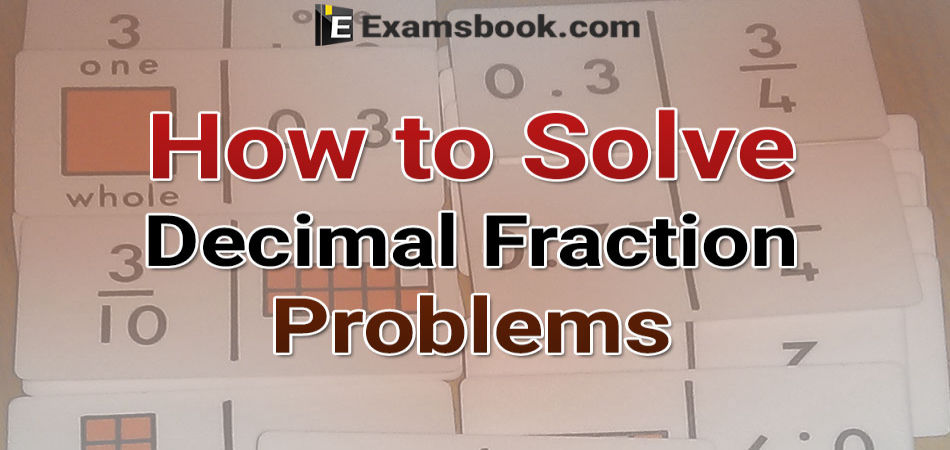• Save

# How to solve decimal fraction problem for SSC and Bank Exams

4 months ago 1.2K ViewsMost of the students are facing problems in decimal fractions in competitive exams. They only think about how they can solve decimal fraction problems. If you also think the same in your mind, you can learn here how to solve decimal fraction problems

In this blog, you can obtain solutions of decimal fraction problems easily. After learning solutions of decimal fraction problems you can practice of aptitude decimal fraction questions and answers also.

You should know the complete detail of decimal fraction with its types.

## How to solve decimal fraction problem in Competitive Exams:

Q.1.  Convert the following into vulgar fractions :

(i) 0.75  (ii) 3.004  (iii) .0056

Solution :

$$(1) \ 0.75= {75\over100}={3\over4}$$

$$(2) \ 3.004= {3.004\over1000}={751\over250}$$

$$(3) \ .0056= {56\over10000}={7\over1250}$$

Q.2.What value will replace the question mark in the following equation  ?

(i) 5172.49+378.352+?=9318.678

(ii) ? – 7328.96 = 5169.38

Solution :

(i) Let 5172.49 + 378.352 + x = 9318.678

Then x = 9318.678 – (5172.49+378.352) = 9318.678 – 5550.842 = 3767.836.

(ii) Let x - 7328.96 = 5169.38. Then, x = 5169.38 + 7328.96 = 12498.34.

Q.3. Find the products : (i) 6.3204 × 100 (ii) .069 × 10000

Solution :

(i) 6.3204 × 100 = 632.04.  (ii) .069 × 10000 = .0690 × 10000 = 690.

Q.4. Find the products :

(i) 2.61 × 1.3   (ii) 2.1693 × 1.4 (iii) .4×.04×.004×40.

Solution :

(i) 261 × 13 = 3393. Sum of decimal places of given numbers = (2+1) = 3.

∴ 2.61 × 1.3 = 3.393.

(ii) 21693 × 14 = 303702. Sum of decimal places = ( 4+1) = 5.

∴ 2.1693 × 1.4 = 3.03702.

(iii) 4 × 4 × 4 × 40 = 2560. Sum of decimal places = (1+2+3) = 6.

∴ .4 × .04 × .004 × 40. = .002560.

Q.5. Given that 268 × 74 = 19832, find the value of 2.68 × .74.

Solution :

Sum of decimal places = (2+2) = 4.

∴ 2.68 × .74 = 1.9832.

Q.6. Find the quotient :

(i) 0.63÷ 9      (ii) 0.0204 ÷ 17  (iii) 3.1603 ÷  13.

Solution :

(i) 63 ÷ 9 = 7. Dividend contains 2 places of decimal.

∴ 0.63 ÷ 9 = .07.

(ii) 204 ÷ 17 = 12. Dividend contains 4 places of decimal.

∴ 0.0204  ÷ 17 = .0012

(iii) 31603  ÷ 13 = 2431. Dividend contains 4 places of decimal.

∴ 3.1603 ÷ 13 = .2431

Q.7. Evaluate :

(i) 35 ÷.07     (ii) 2.5÷ .0005  (iii) 136.09 ÷ 43.9

Solution

$$(1) \ {35\over.07}={35 × 100\over.07× 100}={3500\over7}= 500$$

$$(2) \ {2.5\over0.0005}={2.5×10000\over0.0005×10000}={2500\over5}=5000$$

$$(3) \ {136.09\over43.9}={136.09 × 10\over43.9×10} = {1360.9\over439}=3.1$$

Q.8. What value will come in place of question mark in the following equations ?

(i) 0.006 ÷ ?      (ii) ? + .025 = 80

Solution :

(i) $$Let, \ x={0.006 \over0.6}={0.006 \over0.6}= Then ={0.006 ×10 \over0.6×10}= {0.06 \over6}=0.01$$

(ii) $$Let\ {x\over .025}= 0.80$$

$$Then, \ x= 80 ×.025 = 2.$$

Q.9. 12.1212 + 17.0005 – 9.1102 = ?

Given expression = (12.1212+17.0005) – 9.1102 = (29.1217 – 9.1102) = 20.0115

Q.10. 892.7 – 573.07 – 95.007 = ?

Given expression = 892.7 – (573.07 + 95.007) = 892.7 – 668.077 = 224.623.

These are the solutions of the decimal fraction problems. Students can practice with the help of these solutions in decimal fraction question answers. If you have any doubt regarding how to solve decimal fraction problems, you can ask me in the comment section.